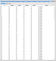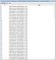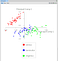# Loading the Dataset

`| dataset f Xmatrix scale X y pca scaled_X reduced_X graph setosa versicolor virginica a b c|f := <path_to_file> asFileReference.dataset := DataFrame readFromCsv: f.dataset columnNames: #( 'slength' 'swidth' 'plength' 'pwidth' 'target' ).dataset removeRowAt: 150.dataset do: [ :row |   row at: #slength transform: [ :element | element asNumber ]. row at: #swidth transform: [ :element | element asNumber ].  row at: #plength transform: [ :element | element asNumber ].  row at: #pwidth transform: [ :element | element asNumber ].     ].`Iris Dataset

# Applying PCA to our Data

`X := dataset columnsFrom: 1 to: 4.y := dataset columnsFrom: 5 to: 5.`
`Xmatrix := PMMatrix rows: ( X asArrayOfRows ).`
`scale := PMStandardizationScaler new.scale fit: Xmatrix.scaled_X := DataFrame withRows: ( (scale fitAndTransform: Xmatrix) rows ) columnNames: #( 'slength' 'swidth' 'plength' 'pwidth' ).Xmatrix := (PMMatrix rows: scaled_X).`
`pca := PMPrincipalComponentAnalyserJacobiTransformation new componentsNumber: 2.pca fit: Xmatrix.Xmatrix := (pca transform: Xmatrix).reduced_X := DataFrame withRows: ( Xmatrix rows ).reduced_X addColumn: (y column: 'target') named: 'target' atPosition: 3.`Iris Data Post PCA

# Visualizing the Data

`a := OrderedCollection new.b := OrderedCollection new.c := OrderedCollection new.(reduced_X) do: [ :row | ( (row at: 'target') = 'Iris-setosa') ifTrue: [ a add: (row asArray )] ].(reduced_X) do: [ :row | ( (row at: 'target') = 'Iris-versicolor') ifTrue: [ b add: (row asArray )] ].(reduced_X) do: [ :row | ( (row at: 'target') = 'Iris-virginica') ifTrue: [ c add: (row asArray )] ].`
`graph := RTGrapher new.setosa := RTData new.versicolor := RTData new.virginica := RTData new.setosa dotShape color: Color red.versicolor dotShape color: Color blue.virginica dotShape color: Color green.setosa points: (a).setosa x: [:vect | vect at: 1].setosa y: [:vect | vect at: 2].setosa label: 'setosa'.versicolor points: (b).versicolor x: [:vect | vect at: 1].versicolor y: [:vect | vect at: 2].versicolor label: 'versicolor'.virginica points: (c).virginica x: [:vect | vect at: 1].virginica y: [:vect | vect at: 2].virginica label: 'virginica'.graph add: setosa.graph add: versicolor.graph add: virginica.graph axisX title: 'Principal Comp 1'.graph axisY title: 'Principal Comp 2'.graph legend below.graph.`Plot

Written by

## Nikhil Pinnaparaju

#### 4th Year Undergraduate Student at the International Institute of Information Technology, Hyderabad

Welcome to a place where words matter. On Medium, smart voices and original ideas take center stage - with no ads in sight. Watch
Follow all the topics you care about, and we’ll deliver the best stories for you to your homepage and inbox. Explore
Get unlimited access to the best stories on Medium — and support writers while you’re at it. Just \$5/month. Upgrade